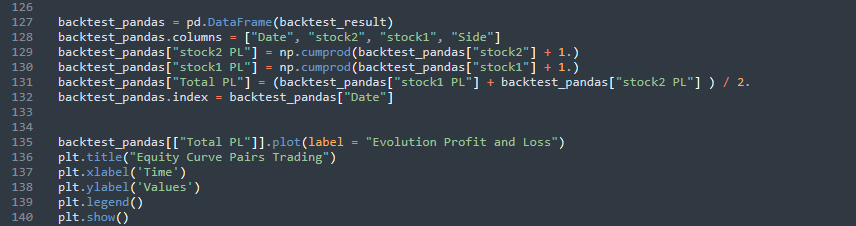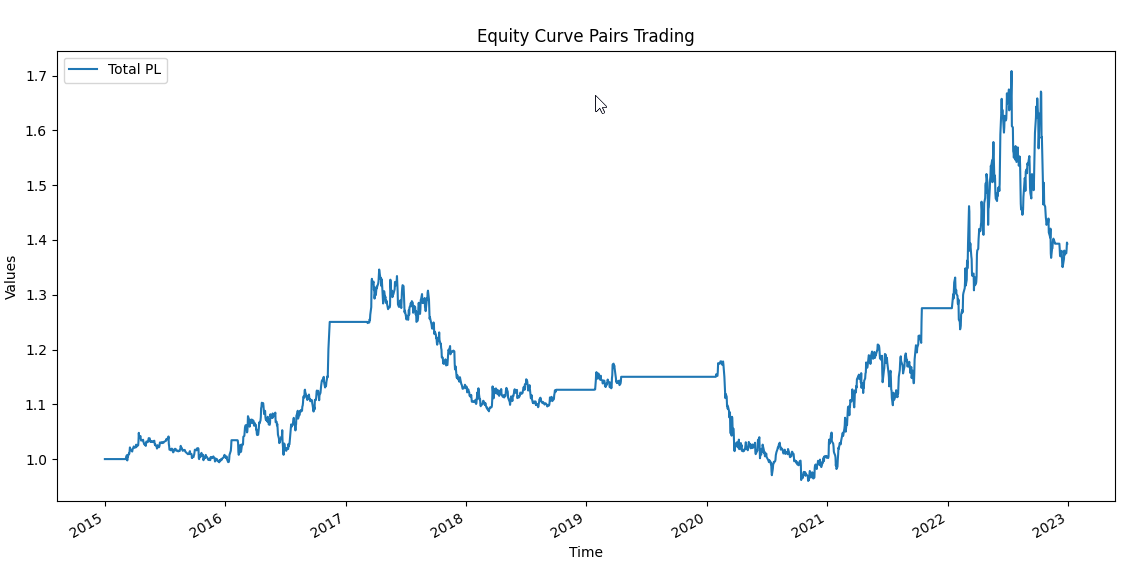# Pairs Trading Strategy With Python (Rules, Example)

Pairs trading strategy is short-term and leverages the Law of One Price, an essential economic concept. This pairs trading strategy with Python involves simultaneously placing two positions in related securities to exploit short-term price divergences.

To illustrate this strategy, consider two imaginary financial instruments, Company A and Company B, whose prices diverge over a specified period. By taking a long position in the underpriced security (Company B) and a short position in the overpriced security (Company A), you can capitalize on the price convergence. This article delves into the intricacies of pairs trading and explores the data visualization of JPMorgan Chase (JPM) and Bank of America (BAC) as a practical example.

Cointegration is a critical aspect of this pairs trading strategy, ensuring a long-term relationship between the two variables. We’ll take you through the steps of testing for cointegration and understanding the results. The z-score, a transformation of the spread, plays a pivotal role in identifying entry and exit points for pairs trading. The article details the calculation of the z-score and setting thresholds to determine when to initiate or close positions.

Furthermore, we discuss the entry and exit rules (trading rules) for pairs trading, providing the necessary Python code for implementation. The article concludes by guiding you through the backtesting process, transforming the results into a data frame, calculating cumulative returns, and visualizing the equity curve. Pairs trading with Python offers a comprehensive approach to profiting from short-term price divergences between related securities.

## Key Takeaways

• This is a short-term strategy where we buy and sell two related things at the same time.
• We do it when their prices don’t match, and we want to make a profit.

The Law of One Price:

• It says that similar things should cost the same in a fair market.
• But sometimes, things can temporarily deviate.

Data for JPMorgan and Bank of America:

• We’re looking at information about two big companies.
• We’re checking how their money and prices have changed over time.

Cointegration:

• We’re checking if two things are connected for a long time.
• It helps us understand if their prices are related.

The Z-score and Signal:

• We use numbers to figure out if prices are acting strange.
• When the numbers go too high or too low, we know something’s up.

Entry and Exit Points:

• We decide when to start and stop buying and selling based on those numbers.

Backtesting:

• We look at how well our plan would have worked in the past to see if it’s a good idea.

## The Law of One Price

Pairs Trading takes a good part of its theory in an essential economic concept, the Law of One Price.

This law says that in an efficient economy, if two commodities or assets are similar, they should have the same price across different locations. This theory assumes that there are no transaction costs, transportation costs, or other costs that influence the final price.

Under this framework, buying one good in one place and reselling it in another region is impossible because producing it in both areas costs the same.

The only possibility to make arbitrage (resell) will be when there is a temporary distortion in the production process, and at that moment, arbitragers will take advantage of this situation.

Pairs trading is a short-term strategy that places two positions simultaneously in two related securities. The idea is to take advantage of the short-term divergence in price between the two financial instruments. Their historical prices need to move together.

The divergence in price will generate a winner and a loser stock. The correct procedure is to place a short position in the winner stock and a long position in the loser stock.

The chart depicts two imaginary financial instruments, Company A and Company B. Both prices diverge from month 6 until month 12. At month 8, we notice that Company A is overpriced and Company B is underpriced, and we expect that the difference in the spread (price Company A – price Company B) will reduce. This scenario leads to the possibility of taking advantage, and we place:

A long position in Company B when the price is $14; subsequently, close the trade when the price arrives at$15.

A short position in Company A when the price is $21; subsequently, close the trade when the price arrives at$17.

The result of this operation is:

### Why the pair divergence?

There are multiple reasons; it can be a natural disaster, corruption scandal, workers on strike, etc. The critical point is that this scenario is temporary.

#### Data Visualization of JPMorgan Chase (JPM) and Bank of America (BAC)

This tutorial will use the financial instruments of JPMorgan Chase (JPM) and Bank of America (BAC). First, let’s see the Return On Equity (ROE):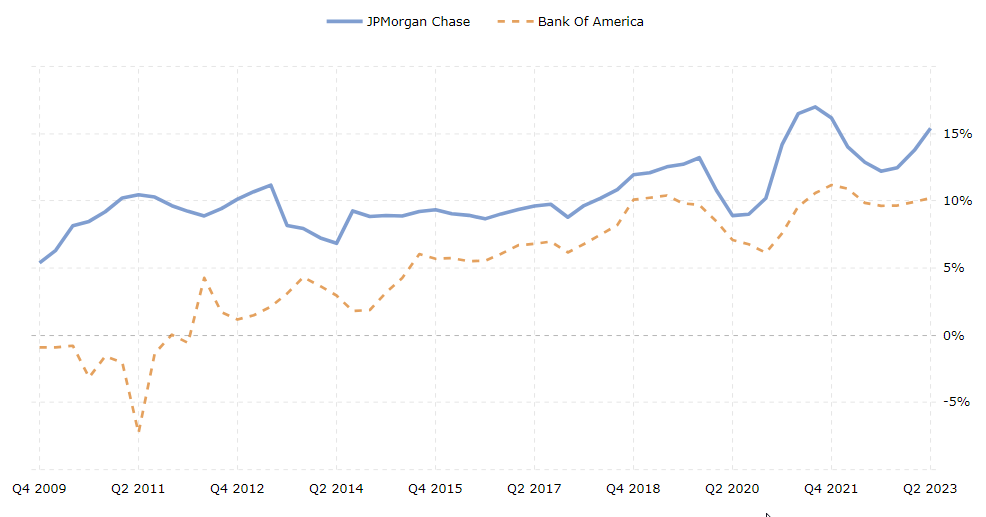Source: macrotrends . net

We can see how the ROE between both companies follows a similar historical pattern. These two institutions are between the largest banks in the U.S. and compete in many areas.

Let’s see their historical price evolution. First, let’s import the Python libraries: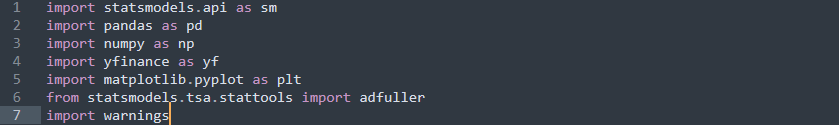Second, download the data with yfinance. The start and end periods are “2015-01-01” and “2023-01-01”: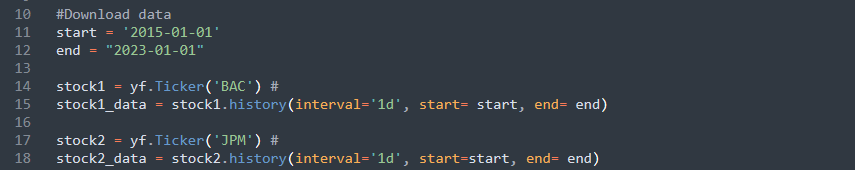Then, we transform the time series , these variables will start from 100. We make this transformation to compare their historical prices: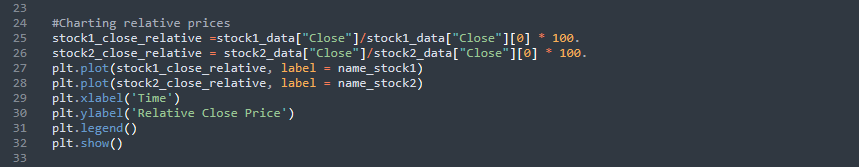Also, in the previous picture is the code for plotting the following chart: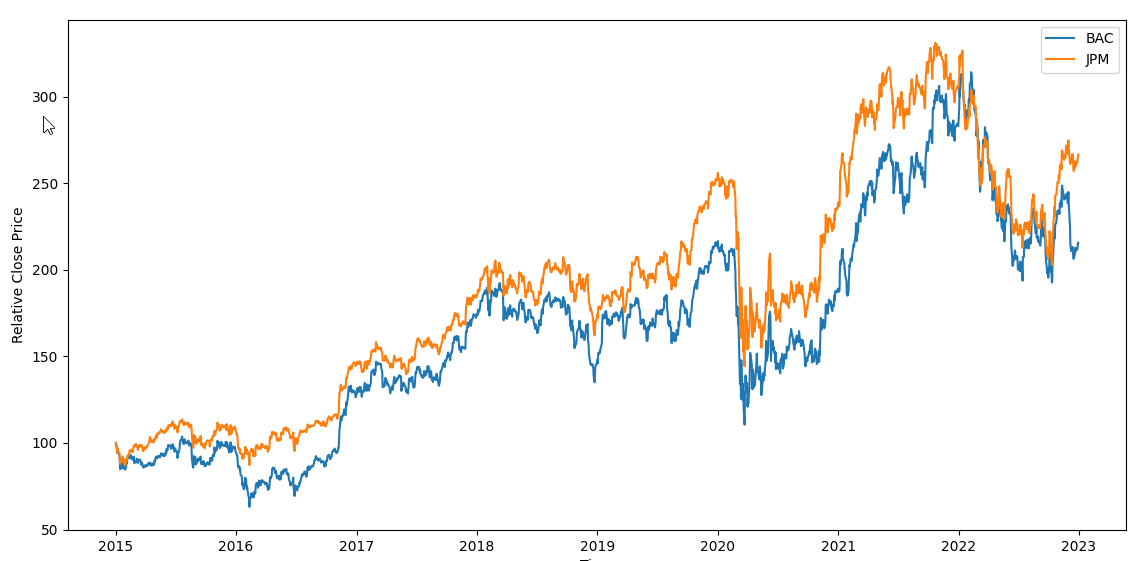The historical prices move in the same direction and follow the same trajectory.

Two variables could move in the same direction, but it does not mean a long-run relationship between them. This phenomenon is called a spurious relationship. The cointegration test tries to find variables with long-run relationships.

The following image has two time series: an integrated order 1 I(1) and an integrated order 0 I(0). The main difference is that the I(0) fluctuates horizontally, and the I(1) can have an upward or downward trend.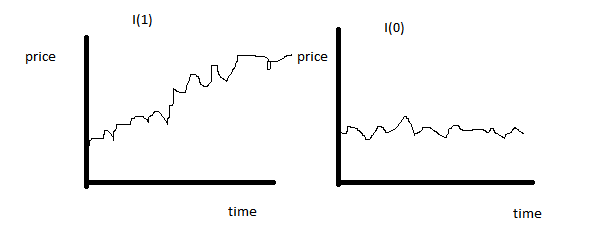If two time series are cointegrated, it means there exists a linear combination of these series of order I(1) that makes the residuals stationary of order I(0).

Step 1

The first step to test cointegration is to estimate a linear regression and get the spread:

The Python code is: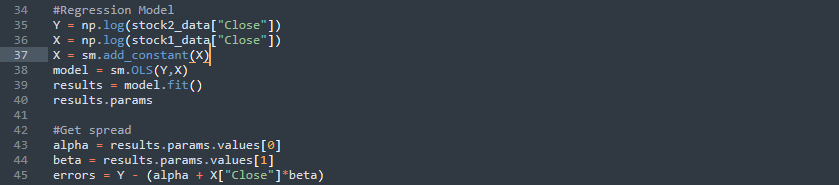In the previous image, we apply algorithms to the closing price; next, we estimate the regression using the Python package statsmodels and its command OLS to get the parameters. Subsequently, we implement to obtain the errors.

Let’s code and see the residuals () plot: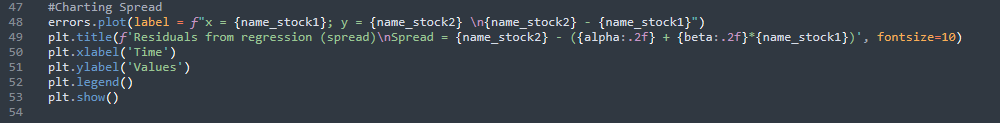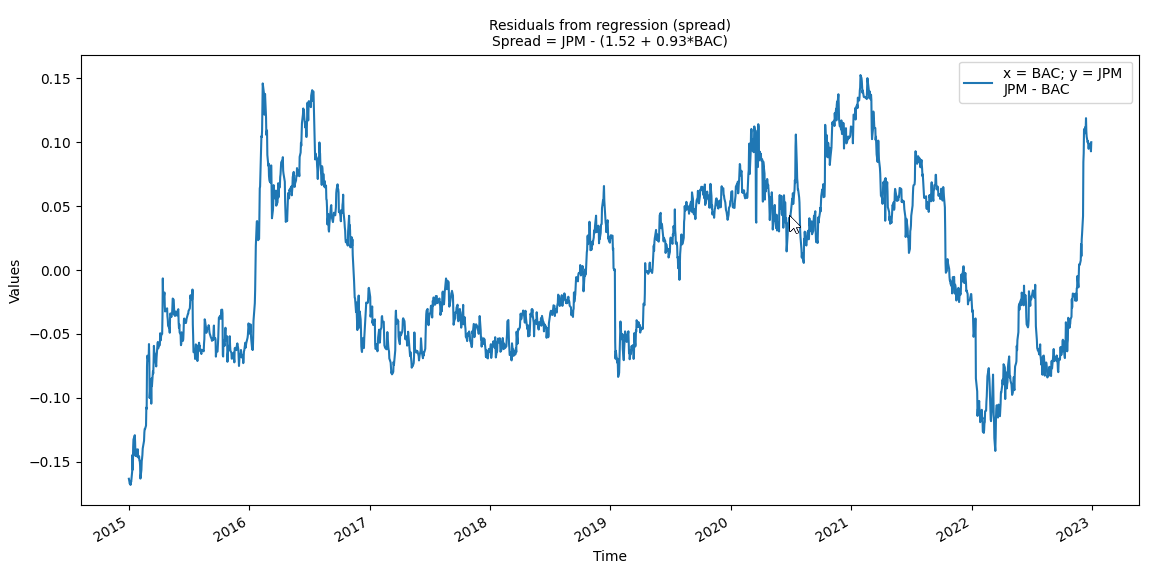In the plot, the errors seem to fluctuate around zero; the values go from -0.16 to 0.16.

Step 2

The second part to test cointegration is to implement the Dickey-Fuller test: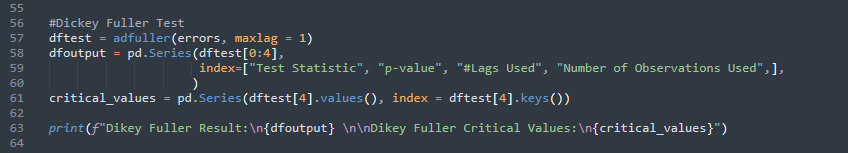The results are in the following image: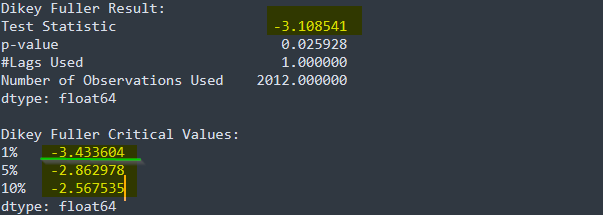Note that the Dickey-Fuller test result -3.108541 lays between the critical values 1% and 5% (see the green horizontal line); therefore, we reject the existence of integrated time series at 10% and 5%, but not at 1%.

If we can not reject the existence of integrated time series, we stop because there is no cointegration between the variables.

## The Z-score and Signal for pairs trading

The z-score is the transformation of the spread. It is calculated as:

We get the residuals (errors) from the previous regression, and we standardized following the previous formula. The code: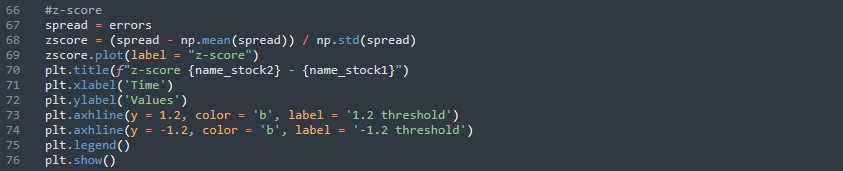Next, we plot the evolution:

The previous chart shows the normalized residuals; they fluctuate around zero, where the minimum and maximum values go from -2.3 to 2.3. Setting thresholds to the z-score in pairs trading is necessary to identify the moment to place operations. Values that are outside those levels indicate that there is a divergence in price between the two financial instruments. The previous chart had ass thresholds 1.2 and -1.2.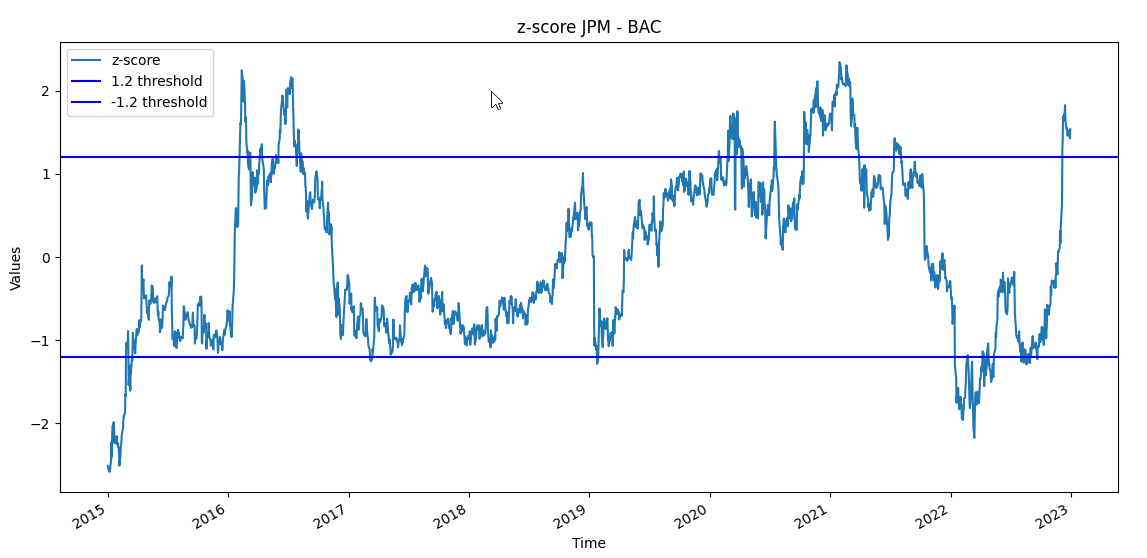We can see that on multiple occasions, every time the z-score is over 1.2 or lower than -1.2, there is a high possibility that the price pull back to zero.

Consider the spread equation , where and . A positive spread is when . It means JPM > BAC; therefore, each time the spread or the z-score is positive, JPM > BAC. Remember, JPM is the winner stock, and BAC is the loser stock.

The entry and exit rules in the strategy are:

Long the spread: place a short position in JPM (winner stock) and a long position in BAC (loser stock) when the z-score crosses 1.2 from below, and there is no position.

Short the spread: place a long position in JPM (loser stock) and a short position in BAC (winner stock) when the z-score crosses 1.2 from above and there is no position.

Exit position: Exit the spread every time the z-score crosses 0, and there is no position.

The code to generate the previous rules is: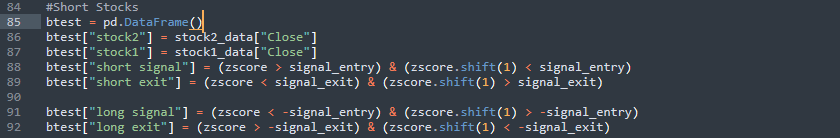The term .shirt(1) generates a time series with the element of the previous day; therefore, It is helpful to check when the z-score crosses the thresholds.

In the following image, you will see the code to generate our backtest: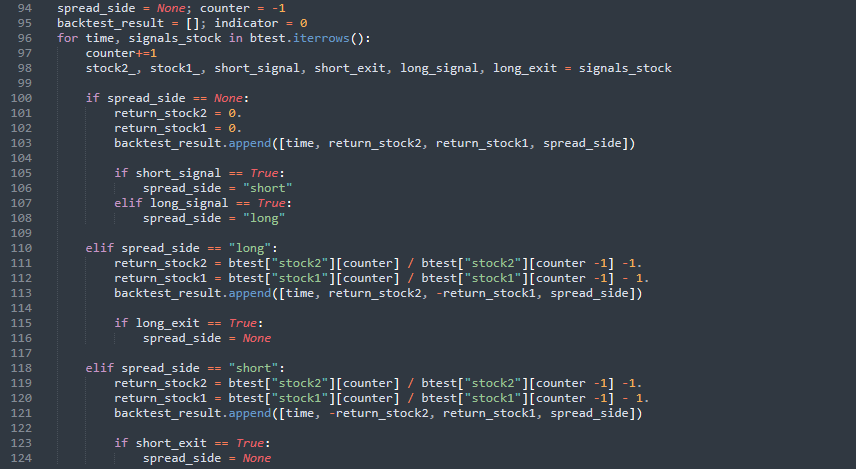Let’s break down the crucial parts. In the following image, the code unpacks the list signals_stock and makes six variables. In case spread_side = None, the returns are zero because there are no positions. Then, if short_signal or long_signal is TRUE, we the value from spread_side.

In case spread_side == “long”, we will calculate the returns and append those results to backtest_result.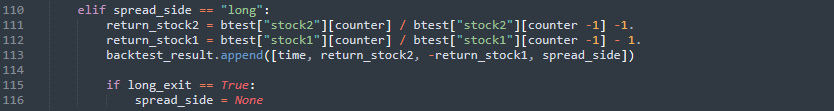In the last condition, spread_side == “short”, the return signs will be inverted: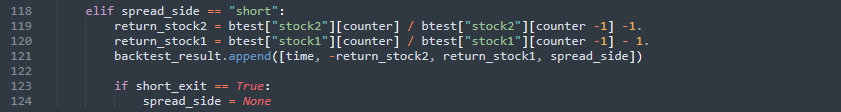The last part of this tutorial is to transform backtest_result in a data frame; next, we calculate the cumulative results and plot the equity curve: